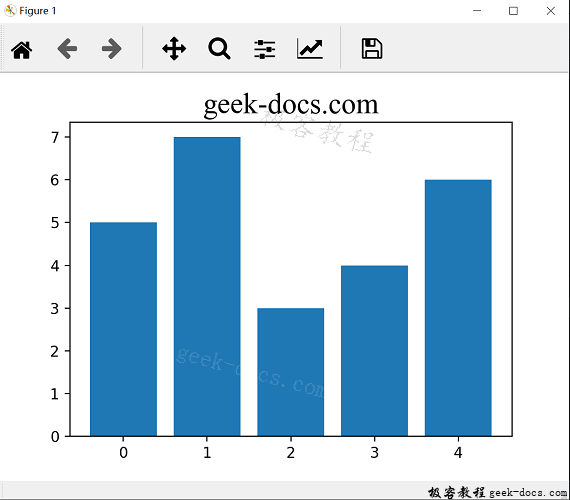# Matplotlib 条形图

Matplotlib 条形图，条形图非常常见，它与直方图极其相似，只不过x轴表示的不是数值而是类别，使用matplotlib的bar()函数就可以生成简单的条形图。

## 绘制条形图

import matplotlib.pyplot as plt

index = [0,1,2,3,4]
values = [5,7,3,4,6]
plt.bar(index, values)
plt.title("geek-docs.com", fontsize=20, fontname="Times New Roman")
plt.show()xticks()函数，作为它的第一个参数。如下所示：

import matplotlib.pyplot as plt

index = [0,1,2,3,4]
values = [5,7,3,4,6]
plt.bar(index, values)
plt.title("geek-docs.com", fontsize=20, fontname="Times New Roman")
plt.xticks(index,['A', 'B', 'C', 'D', 'E'])
plt.show()## 添加图例

• error_kw：用于接收其他可用于显示误差线的关键字参数。eColor参数指定误差线的颜色，capsize参数指定误差线两头横线大的宽度。
• alpha：控制彩色条形图的透明度，取值范围是0-1，0表示完全透明，随alpha的增加，对象逐渐清晰起来，到1时不透明。
• label：为图表中的序列指定名称。
import matplotlib.pyplot as plt
import numpy as np

index = np.arange(5)
values = [5,7,3,4,6]
std1=[0.8, 1, 0.4, 0.9, 1.3]
plt.title("geek-docs.com", fontsize=20, fontname="Times New Roman")
plt.bar(index, values, yerr=std1,error_kw={'ecolor':'0.1', 'capsize':6}, alpha=0.7, label='First')
plt.xticks(index,['A', 'B', 'C', 'D', 'E'])
plt.legend(loc=2)
plt.show()• 回顶